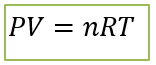# Problem: Complete the following table for an ideal gas: P  V  n  T 2.10 atm  1.30 L0.510 mol? K0.320 atm0.260 L? mol32 oC660 torr? L0.334 mol360 K? atm585 mL0.260 mol290 KComplete the third row of the table.

###### FREE Expert Solution

The mathematical representation of the Ideal Gas Law is:where P is pressure in atm

V is volume in L

n is number of moles

R is the universal gas constant (0.08206 L∙atm/mol∙K)

T is temperature in K

92% (258 ratings)###### Problem Details

Complete the following table for an ideal gas:

 P V n T 2.10 atm 1.30 L 0.510 mol ? K 0.320 atm 0.260 L ? mol 32 oC 660 torr ? L 0.334 mol 360 K ? atm 585 mL 0.260 mol 290 K

Complete the third row of the table.